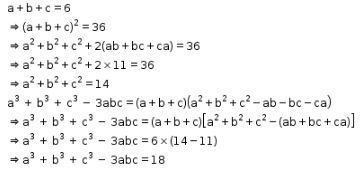# If a + b + c = 6 and ab + bc + ca = 11, find the value of a^3 + b^3 + c^3 - 3abc

If a + b + c = 6 and ab + bc + ca = 11, find the value of a3 + b3 + c3 - 3abc